Vol. Practical Guide to Radio-Frequency Analysis and Design

# The Many Types of Radio Frequency Modulation

## RF communication is built upon a simple concept: by continually altering the characteristics of a sinusoid, we can use it to transfer information.

RF communication is built upon a simple concept: by continually altering the characteristics of a sinusoid, we can use it to transfer information.

At this point, we have covered a variety of important concepts that serve as a foundation for the successful design and analysis of real-world RF circuits and systems. We are now ready to explore a fundamental aspect of RF engineering: modulation.

### What Is Modulation?

The general meaning of the verb “to modulate” is “to modify, to regulate, to vary,” and this captures the essence of modulation even in the specialized context of wireless communication. To modulate a signal is simply to intentionally modify it, but of course, this modification is done in a very specific way because the goal of modulation is data transfer.

We want to transfer information—ones, and zeros if we’re dealing with digital data, or a sequence of continuously varying values if we are working in the analog realm. But the restrictions imposed by wireless communication do not allow us to express this information in the typical way; instead, we have to devise a new “language,” or you might think of it as a code, that allows us to convey the same information but within the constraints of an electromagnetic-radiation-based system. More specifically, we need a language that is compatible with high-frequency sinusoidal signals, because such signals constitute the only practical means of “carrying” information in a typical RF system.

This high-frequency sinusoid that is used to carry information is called, appropriately, the carrier. It’s a helpful name because it reminds us that the purpose of an RF system is not to generate and transmit a high-frequency sinusoid. Rather, the purpose is to transfer (lower-frequency) information, and the carrier is simply the means that we must use to move this information from an RF transmitter to an RF receiver.

### Modulation Schemes

In verbal communication, the human body generates sound waves and modifies—or modulates—them so as to produce a wide variety of vowels and consonants. Intelligent use of these vowels and consonants results in the transfer of information from the speaker to the listener. The system according to which the sound waves are modulated is called a language.

In RF communication, the situation is very similar. A device modulates electrical waves according to a predefined system called a modulation scheme (or modulation technique). Just as there are many human languages, there are many ways in which a carrier can be modulated.##### Sophisticated modulation schemes help modern RF systems to achieve increased range and improved immunity to interference.

It is possible that certain human languages are especially effective in conveying certain types of information; to take an example from the ancient world, perhaps Greek was better for philosophy and Latin was better for codifying laws. There is no doubt, however, that reliable communication is possible with any properly developed language, as long as the speaker and the listener both know it. The same is true for RF systems. Each modulation scheme has its advantages and disadvantages, but all can provide excellent wireless communication if the fundamental requirement is fulfilled—i.e., the receiver must be able to understand what the transmitter is saying.

### Amplitude, Frequency, Phase

A basic sinusoid is a simple thing. If we ignore DC offset, it can be completely characterized with only two parameters: amplitude and frequency. We also have phase, which comes into play when we consider the initial state of the sinusoid, or when changes in wave behavior allow us to contrast one portion of the sinusoid with a preceding portion. Phase is also relevant when comparing two sinusoids; this aspect of sinusoidal phase has become very important because of the widespread use of quadrature (or “IQ”) signals in RF systems. We’ll look at IQ concepts later in the textbook.

As discussed above, modulation is modification, and we can modify only what is already present. Sinusoids have amplitude, frequency, and phase, and thus it should come as no surprise that modulation schemes are categorized as amplitude modulation, frequency modulation, or phase modulation. (Actually, it is possible to bridge these categories by combining amplitude modulation with frequency or phase modulation.) Within each category we have two subcategories: analog modulation and digital modulation.

#### Amplitude Modulation (AM)

Analog AM consists of multiplying a continuously varying sinusoidal carrier by an offset version of a continuously varying information (aka baseband) signal. By “offset version” I mean that the amplitude of the baseband signal is always greater than or equal to zero.

Let’s assume that we have a 10 MHz carrier and a 1 MHz baseband waveform: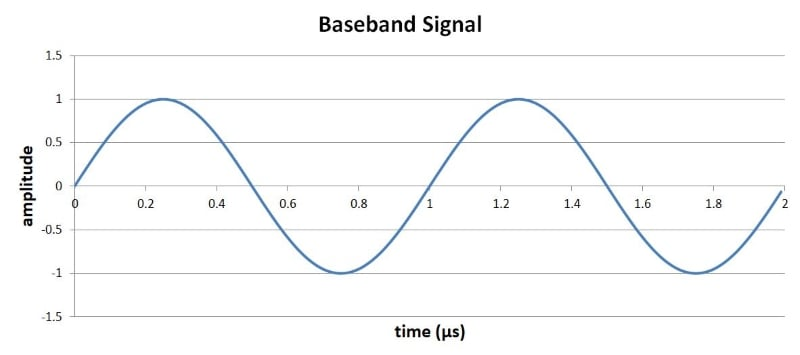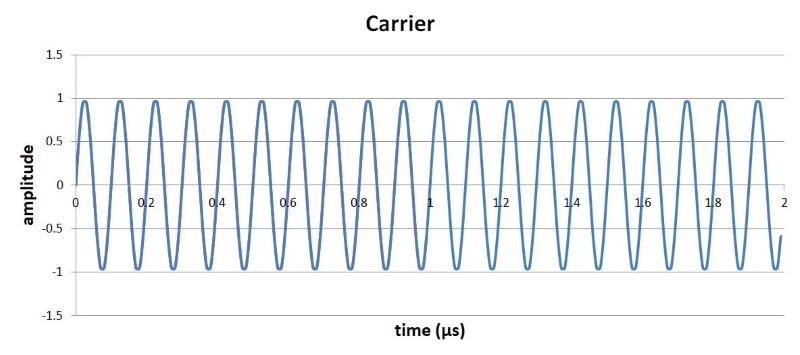If we multiply these two signals, we get the following (incorrect) waveform: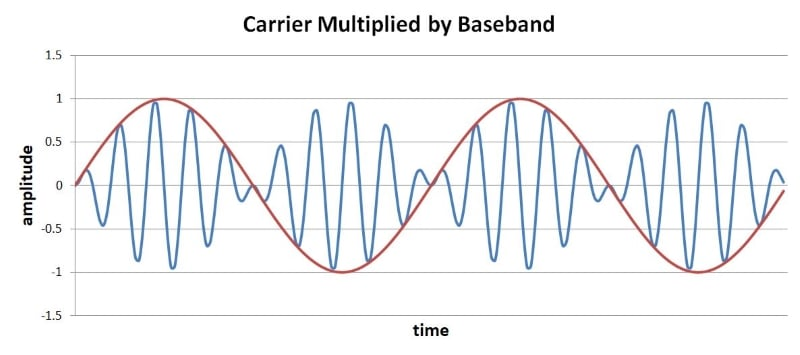You can clearly see the relationship between the baseband signal (red) and the amplitude of the carrier (blue).

But we have a problem: If you look only at the amplitude of the carrier, how can you determine if the baseband value is positive or negative? You can’t—and, consequently, amplitude demodulation will not extract the baseband signal from the modulated carrier.

The solution is to shift the baseband signal so that it varies from 0 to 2 instead of -1 to 1: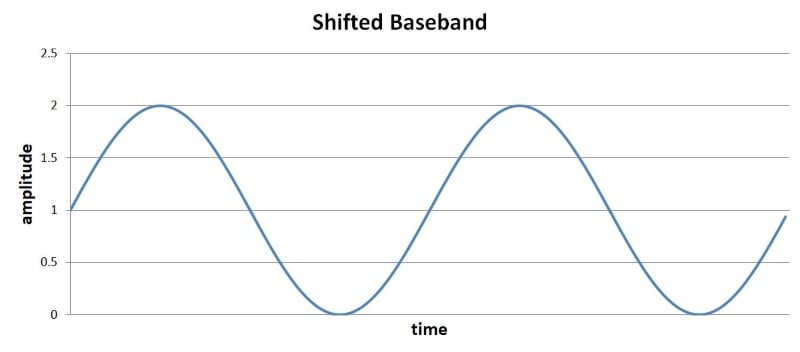If we multiply the shifted baseband signal by the carrier, we have the following: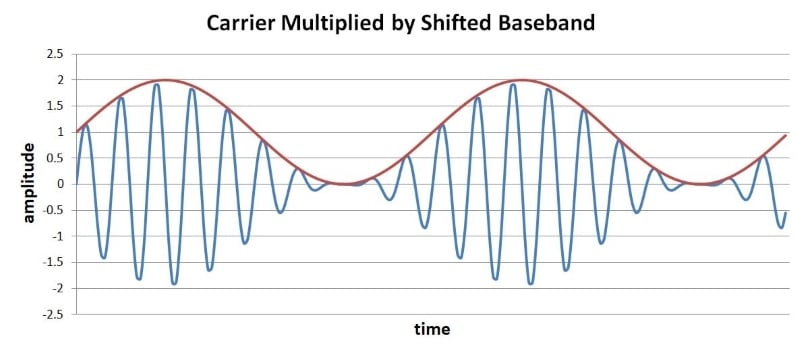Now the amplitude of the carrier can be mapped directly to the behavior of the baseband signal.

The most straightforward form of digital AM applies the same mathematical relationship to a baseband signal whose amplitude is either 0 or 1. The result is referred to as “on-off keying” (OOK): when the information signal is logic zero, the carrier’s amplitude is zero (= “off”); when the information signal is logic one, the carrier is at full amplitude (= “on”).

#### Frequency Modulation (FM) and Phase Modulation (PM)

FM and PM are closely related because frequency and phase are closely related. This is not so obvious if you think of frequency as the number of full cycles per second—what does cycles per second have to do with the position of the sinusoid at a given moment during its cycle? But it makes more sense if you consider the instantaneous frequency, i.e., the frequency of a signal at a given moment. (It is undoubtedly paradoxical to describe a frequency as instantaneous—but, in the context of practical signal processing, we can safely ignore the complicated theoretical details associated with this concept.)

In a basic sinusoid, the value of the instantaneous frequency is the same as that of the “normal” frequency. The analytical value of instantaneous frequency arises when we are dealing with signals that have a time-varying frequency, i.e., the frequency is not a constant value but rather a function of time, written as ω(t). In any event, the important point for our current discussion regarding the close relationship between frequency and phase is the following: instantaneous angular frequency is the derivative, with respect to time, of phase. So if you have an expression φ(t) that describes the time-varying behavior of the signal’s phase, the rate of change (with respect to time) of φ(t) gives you the expression for instantaneous angular frequency:

$$\omega(t)=\frac{\text{d}\phi(t)}{\text{d}t}$$

We’ll take a closer look at frequency and phase modulation later in this chapter. For now let’s conclude with the following plot, which applies the mathematical relationship for frequency modulation to the baseband and carrier signals used above: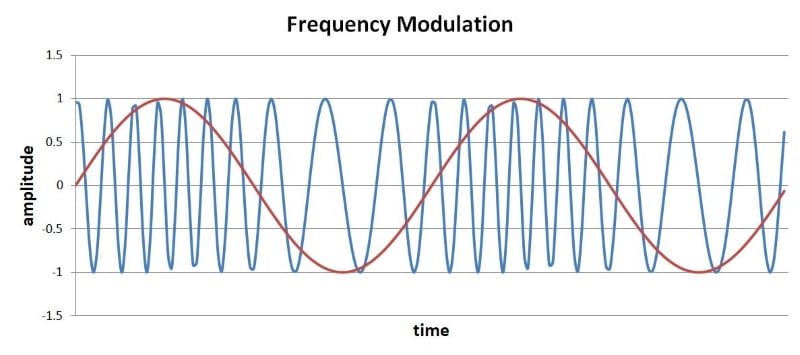### Summary

• Modulation refers to the process of carefully modifying an existing signal so that it can transfer information.
• In the context of RF, the existing signal is called the carrier, and the information is contained in the baseband signal.
• There are many different modulation schemes, meaning that there are different ways of incorporating baseband information into a sinusoidal carrier wave.
• Modulation involves modification of a carrier’s amplitude, frequency, or phase, and it can be used to transfer analog signals or digital data.

#### Lessons in Electric Circuits

Published under the terms and conditions of the Design Science License•chrislafave February 18, 2020